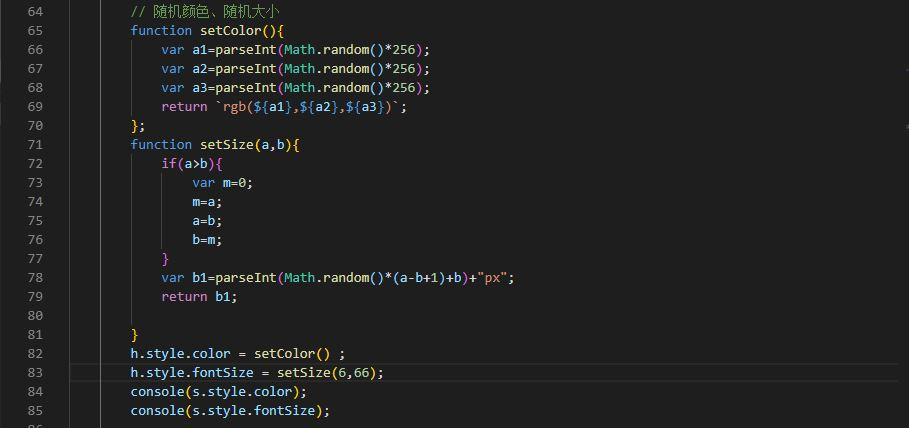# JS13-Math方法

<span id="s">我要执行随机字体颜色</span>

<div id="d">我要改变背景颜色</div>

<script>


Math方法,是JavaScript提供的方法,可以直接使用

1, 随机数 Math.random()

2.应用:设定随机颜色等

rgb(数值1,数值2,数值3)

var c1 = parseInt(Math.random()*256);
var c2 = parseInt(Math.random()*256);
var c3 = parseInt(Math.random()*256);


JavaScript中DOM操作,操作标签样式的固定语法

s.style.color = rgb(${c1},${c2},${c3});  生成随机颜色的程序,写成函数的形式 这个函数的返回值是 rgb(随机颜色) 字符串 颜色的数值范围是固定的 0 - 255 function setColor(){ var c1 = parseInt(Math.random()*256); var c2 = parseInt(Math.random()*256); var c3 = parseInt(Math.random()*256); // 将随机颜色,定义为rgb字符串,作为返回值 // 作为设定颜色的属性值 return rgb(${c1},${c2},${c3});
}

// 定义随机大小函数
// 定义两个参数,a是较小值,b是较大值
// 通过参数,设定随机数值范围
function setNum(a,b){
// 做容错处理,确保 a < b
if(a>b){
var m = 0;
m = a;
a = b;
b = m;
}
// 随机数值要拼接px单位
return parseInt(Math.random()*(b+1-a) + a) + 'px';
}3.定时器不用掌握,让你们瞅瞅的内容

setInterval( function(){要执行的程序} , 时间 )

setInterval( function(){
// 改变id是s,也就是span标签的字体颜色
s.style.color = setColor() ;
// 字体大小,JavaScript中,不支持 减号 font-size
// font-size 必须写成 fontSize 驼峰命名法
// 所有带有 -减号的属性值,都要写成小驼峰命名法
s.style.fontSize = setNum(50,100);

// 改变id是d,也就是div标签的背景颜色
d.style.background = setColor() ;

// 定义div标签的宽度高度,并且设定范围
d.style.width = setNum(200,300);
d.style.height = setNum(200,300);

} , 1000)

</script>©️2019 CSDN 皮肤主题: 深蓝海洋 设计师: CSDN官方博客